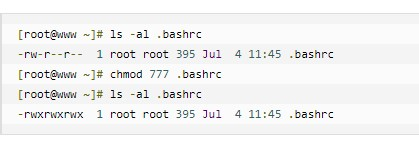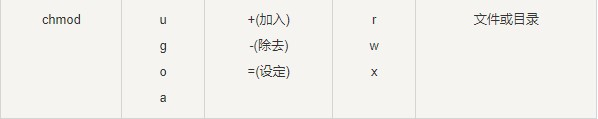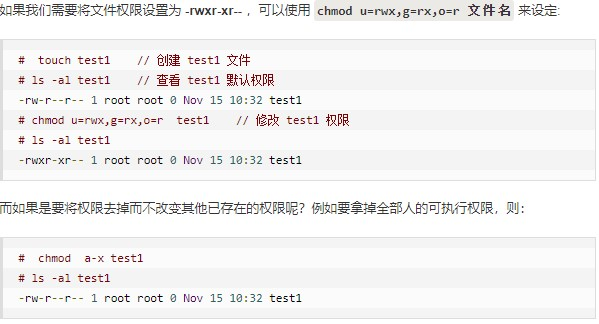] 分数则是：

• owner = rwx = 4+2+1 = 7
• group = rwx = 4+2+1 = 7
• others= --- = 0+0+0 = 0
##### 5.2.3.1 使用数字修改权限
• chmod [-R] xyz 文件或目录
• xyz : 就是刚刚提到的数字类型的权限属性，为 rwx 属性数值的相加。
• -R : 进行递归(recursive)的持续变更，亦即连同次目录下的所有文件都会变更
• 举例来说，如果要将.bashrc这个文件所有的权限都设定启用，那么命令如下：##### 5.2.3.1 使用符号修改权限
• 我们就可以使用 u(user), g(group), o(others) 来代表三种身份的权限！
• 此外， a 则代表 all，即全部的身份。读写的权限可以写成 r, w, x，也就是可以使用下表的方式来看• 举例说明